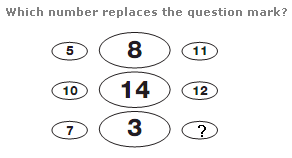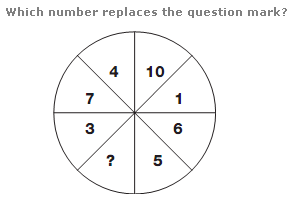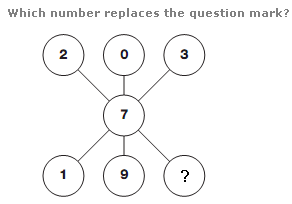# Puzzles - Number puzzles

Exercise : Number puzzles
100.:
4
Explanation
:
In each row of the diagram, the sum of the left and right hand digits, minus the central digit is always 8.

101.:
8
Explanation
:
Divide the circle, horizontally and vertically, to give 4 quarters. The sum of the numbers in the segments of each quarter equals 11.

102.